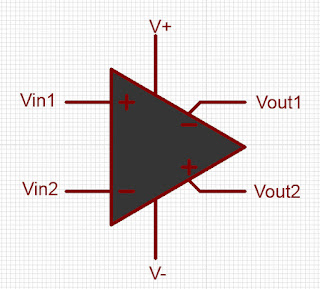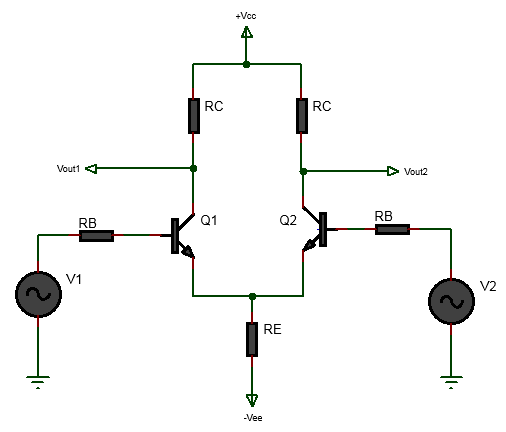# Differential and Common Mode Operation of BJT Differential Amplifier

Differential amplifiers are a fundamental building block of electronic circuits, used in a wide variety of applications, from signal amplification to filtering, and is used as part in many electronics circuits such as differential amplifier modulator, voltage controlled oscillator, and is the basic building block in operational amplifier. One of the key features of differential amplifiers is their ability to perform differential and common-mode operations. Differential amplifier are typically implemented in integrate circuit chips but can also be implemented with discrete individual BJT or MOSFET or FET or combination of these transistors such as BiCMOS technology. In this blog post, we will explore the differential and common mode operation of a BJT differential amplifiers in more detail.

## What is a Differential Amplifier?

Before we dive into the differential and common-mode operation of differential amplifiers, let's first define what a differential amplifier is. A BJT differential amplifier is a type of amplifier that amplifies the difference between two input signals. The following shows a differential amplifier block diagram.Internally, it is implemented using a pair of identical transistors(Q1,Q2), configured in a differential pair, with identical biasing resistors(RC,RB,RE) as shown in the figure below.The above circuit implementation of differential amplifier is called dual input balanced output topology. This topology is called dual input because there are two inputs V1 and V2 and balanced output because the output is taken between the two outputs Vout1 and Vout2. We can also have other topology which is described in the types of differential amplifier and how they work.

### Mode of Operation of Differential Amplifier Circuit

Basically, the differential amplifier amplifies the voltage difference between the two input signals, v1 and v2, and produces an output voltage, vout, which is proportional to the difference between v1 and v2. The gain of the differential amplifier is determined by the properties of the transistors and the biasing of the circuit. However it's operation should be understood for two mode of operations, namely,

(a) Differential Mode Operation

(b) Common Mode Operation

These two mode of operation of BJT differential amplifier are explained next.

### Differential Mode Operation

Differential mode operation refers to the situation where the input signals v1 and v2 are equal in magnitude but opposite in polarity. The following shows the circuit diagram of operation of BJT amplifier in differential mode of operation.

In the circuit diagram above, two equal and opposite phase input signals is achieved using a transformer. These two equal and opposite phase signals are applied to the bases of the two differential pair transistors Q1 and Q2.

In this case, the differential amplifier amplifies the voltage difference between the two signals, which is twice the amplitude of each individual signal. The output voltage, $$vout$$, is given by the following equation:

$$V_{out} = A_{dm}(V_1-V_2)$$

where $$A_{dm}$$ is the differential gain of the amplifier.

The difference between the two input signals is called differential input signal.

$$V_d = V1-V2$$

and hence we can also write,

$$V_{out} = A_{dm}V_d$$

Differential mode operation is useful for applications such as amplifying small signals, rejecting common-mode noise, and subtracting two signals.

The following shows waveform of the two input signals V1, V2 which are of equal magnitude but are out of phase and the differential signal Vd taken between Vout1 and Vout2.

The applied input signals V1 and V2 was 50mVpk with opposite phase.

The following shows how the 2N3904 BJT differential amplifier operates in differential mode.

### Common Mode Operation

Common mode operation refers to the situation where the input signals v1 and v2 are equal in magnitude and polarity. In this case, the differential amplifier amplifies the common-mode voltage, which is the average of the two input signals. The output voltage, vout, is given by the following equation:

$$V_{out} = A_{cm}(V_{cm})$$

where $$A_{cm}$$ is the common-mode gain of the amplifier.

where the common mode $$V_{cm}$$ is defined as,

$$V_{cm} = \frac{V_1+V_2}{2}$$

Common mode operation is useful for applications such as sensing the level of a signal relative to ground or rejecting differential noise.

With 50mVpk voltage for V1 and V2 which are both inphase, the  waveform of the two inputs and the common mode signal is shown below.

The following shows the common mode signal $$V_{cm}$$

The following video demonstrates common mode operation of 2N3904 BJT differential amplifier.

#### Common Mode Rejection Ratio

One of the key advantages of differential amplifiers is their ability to reject common-mode signals while amplifying differential signals. The degree to which a differential amplifier rejects common-mode signals is measured by the common-mode rejection ratio (CMRR). The CMRR is defined as the ratio of the differential gain to the common-mode gain as follows:

$$CMRR = \frac{A_{dm}}{A_{cm}}$$

CMRR is often expressed in decibels (dB) as follows,

$$CMRR(dB)=20log_{10}(\frac{A_{dm}}{A_{cm}})$$

Another important parameter for differential amplifiers is the differential mode rejection ratio (DMRR), which measures the degree to which the amplifier rejects differential signals. The DMRR is defined as the ratio of the common-mode gain to the differential gain, also expressed in decibels.

Conclusion

In conclusion, differential amplifiers are a versatile building block of electronic circuits that can perform both differential and common-mode operations. Differential mode operation amplifies the voltage difference between two input signals, while common mode operation amplifies the average voltage of the two signals. The ability of differential amplifiers to reject common-mode signals while amplifying differential signals makes them useful in a wide variety of applications. The CMRR and DMRR are important parameters that characterize the performance of differential amplifiers.

References: